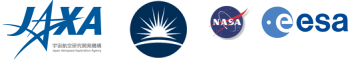## Evidence that Waves Heat Coronal Holes

Michael Hahn and Daniel Wolf Savin (Columbia Astrophysics Laboratory, Columbia University)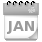Using EIS observations of a coronal hole, we have found that Alfvén waves not only carry enough energy to heat the plasma and drive the fast solar wind, but also that this energy is dissipated at low enough heights for it to thermalize throughout the coronal hole. Alfvén waves have long been proposed as the energy source that heats the corona and drives the solar wind. But a challenge for wave-driven models has been to show that the waves damp at low enough heights in the corona. In order to heat the corona, the waves must be damped below about 2 R, that is, below the heights where heat conduction becomes inefficient and the solar wind becomes supersonic.

Spectral line widths were used to infer the amplitude of the waves, which is proportional to the non-thermal line broadening, expressed as a velocity vnt. The energy flux carried by the waves can be derived from the amplitude and damping can be seen in the variation of the line widths verus height. There are several systematic issues with this method that have to be addressed.

One problem is to account for instrument scattered light. Line widths observed on the disk are narrower than in the off-disk corona. Thus, if scattered light significantly contaminates the off-disk spectrum, it can cause the lines to appear narrower.

We have quantified the instrument scattered light by measuring the intensities of lines that are expected to be weak off-limb but are strong on the disk. For example He II, O IV, O V, and O VI are transition region lines and should be weak in the corona. We determined that their intensity at large heights was about 2% or less of the intensity in the on-disk portions of our observation. We therefore corrected for scattered light in our measurements by subtracting Gaussian model stray light line profiles with intensities equal to 2% of the on-disk intensity, and line widths and centroid wavelengths the same as on the disk. More details about quantifying and correcting for stray light can be found in the references.Figure 1: Intensity of lines from O IV, V, and VI as a function of height. The intensity of these lines drops rapidly at the limb and is consistent with zero at large heights. This is one indication that the instrument scattered light level at large heights is small.

Another challenge for determining vnt is to seperate out the thermal broadening. The thermal broadening is proportional to the ion temperature, Ti. It is sometimes assumed that Ti is equal to the electron temperature, but observations have shown that this is not the case for coronal holes. To measure both Ti and vnt we used a different approach. First, we assume that Ti is constant with height, so that any changes in the line width are due only to changes in vnt. Second, we assume that at the lowest heights, up to about 1.1 R, the waves are undamped. This has been found by a number of previous observations. If the waves are undamped, then vnt is proportional to the electron density as ne-1/4, which can be measured independently. These assumptions provide enough constraints to determine Ti and vnt.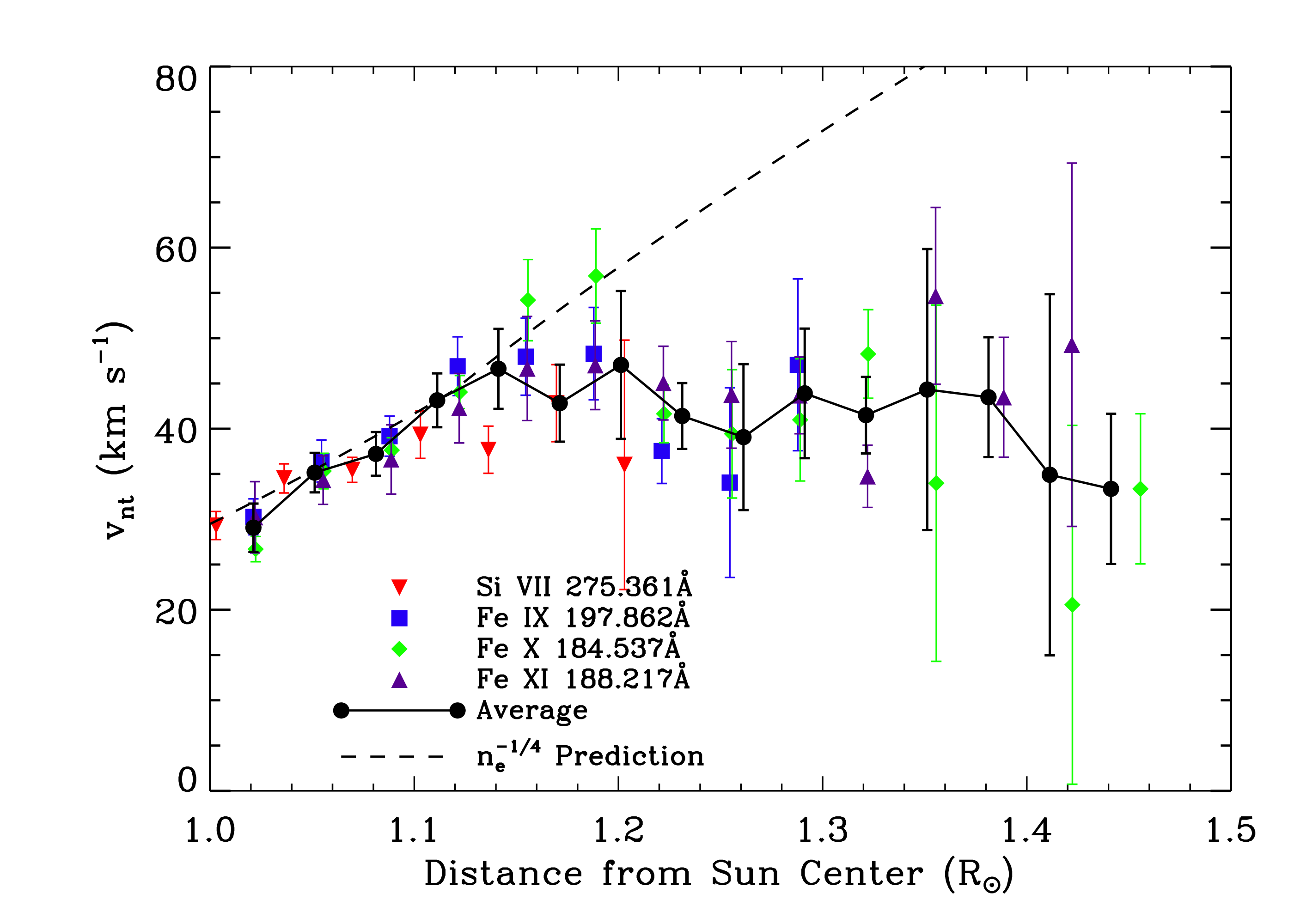Figure 2: Colored symbols show vnt versus height for several different lines. The solid line indicates the average of these points. The dashed line shows the ne-1/4 trend that is predicted for undamped waves. The measurements imply that at about 1.15 R the waves begin to be strongly damped.

From the derived vnt versus height (Figure 2), we estimate the energy flux of the waves and some characteristics of the damping. Figure 3 plots the energy flux F after correcting for the geometrical expansion of the magnetic flux tubes as the waves propagate. We find that the amount of wave energy flux at the base of the corona is about 6.7 x 105 erg cm-2 s-1. We estimate that about 6 x 105 erg cm-2 s-1 of energy is required to heat the observed coronal hole and accelerate the fast solar wind. Thus, the energy carried by the waves is sufficient to power the coronal hole. Moreover, we find that this energy is damped over a length scale of about 0.18 R.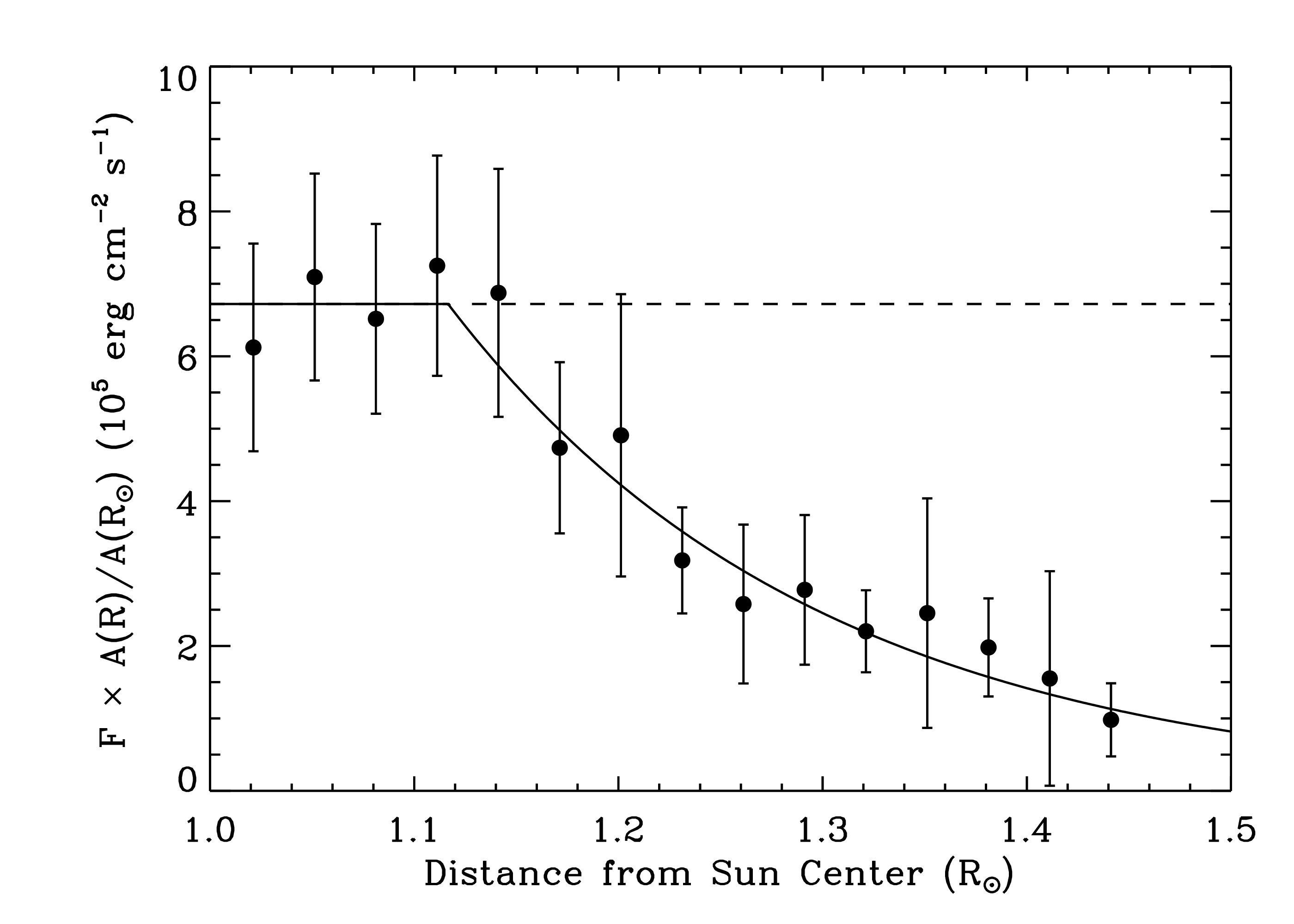Figure 3: The filled circles show the wave energy flux, F, corrected for the area expansion. If the waves were undamped the points would fall on the straight horizontal line indicated by the dashed line. The solid curve is a fit used to estimate some characteristics of the waves and the damping. This function is initially constant and later has an exponential decay.

In summary, our measurements of EIS line widths demonstrate that Alfvén waves carry and dissipate enough energy at sufficiently low heights to heat coronal holes and accelerate the fast solar wind.

References
Hahn, M., Landi, E., and Savin, D. W., 2012, ApJ, 753, 36.
Hahn, M., and Savin, D. W., 2013, ApJ, 776, 78.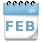Next EIS Nugget    »»  coming later...

TBC

Last Revised: 26-Sep-2013